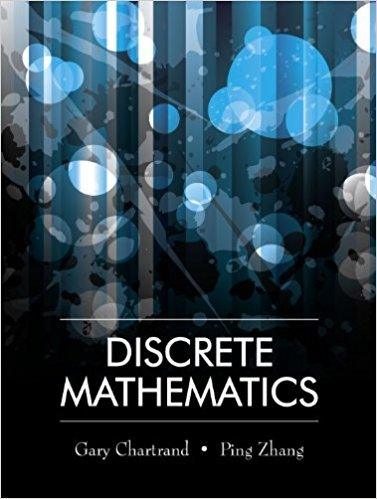×
×

# Solutions for Chapter 7.5: Introduction to Cryptography## Full solutions for Discrete Mathematics | 1st Edition

ISBN: 9781577667308Solutions for Chapter 7.5: Introduction to Cryptography

Solutions for Chapter 7.5
4 5 0 357 Reviews
18
0
##### ISBN: 9781577667308

Since 11 problems in chapter 7.5: Introduction to Cryptography have been answered, more than 13905 students have viewed full step-by-step solutions from this chapter. This expansive textbook survival guide covers the following chapters and their solutions. Discrete Mathematics was written by and is associated to the ISBN: 9781577667308. This textbook survival guide was created for the textbook: Discrete Mathematics, edition: 1. Chapter 7.5: Introduction to Cryptography includes 11 full step-by-step solutions.

Key Math Terms and definitions covered in this textbook
• Change of basis matrix M.

The old basis vectors v j are combinations L mij Wi of the new basis vectors. The coordinates of CI VI + ... + cnvn = dl wI + ... + dn Wn are related by d = M c. (For n = 2 set VI = mll WI +m21 W2, V2 = m12WI +m22w2.)

• Cyclic shift

S. Permutation with S21 = 1, S32 = 1, ... , finally SIn = 1. Its eigenvalues are the nth roots e2lrik/n of 1; eigenvectors are columns of the Fourier matrix F.

• Diagonal matrix D.

dij = 0 if i #- j. Block-diagonal: zero outside square blocks Du.

• Diagonalizable matrix A.

Must have n independent eigenvectors (in the columns of S; automatic with n different eigenvalues). Then S-I AS = A = eigenvalue matrix.

• Echelon matrix U.

The first nonzero entry (the pivot) in each row comes in a later column than the pivot in the previous row. All zero rows come last.

• Free variable Xi.

Column i has no pivot in elimination. We can give the n - r free variables any values, then Ax = b determines the r pivot variables (if solvable!).

• Full row rank r = m.

Independent rows, at least one solution to Ax = b, column space is all of Rm. Full rank means full column rank or full row rank.

• Graph G.

Set of n nodes connected pairwise by m edges. A complete graph has all n(n - 1)/2 edges between nodes. A tree has only n - 1 edges and no closed loops.

• Hilbert matrix hilb(n).

Entries HU = 1/(i + j -1) = Jd X i- 1 xj-1dx. Positive definite but extremely small Amin and large condition number: H is ill-conditioned.

• Jordan form 1 = M- 1 AM.

If A has s independent eigenvectors, its "generalized" eigenvector matrix M gives 1 = diag(lt, ... , 1s). The block his Akh +Nk where Nk has 1 's on diagonall. Each block has one eigenvalue Ak and one eigenvector.

• Kirchhoff's Laws.

Current Law: net current (in minus out) is zero at each node. Voltage Law: Potential differences (voltage drops) add to zero around any closed loop.

• Left nullspace N (AT).

Nullspace of AT = "left nullspace" of A because y T A = OT.

• Linear combination cv + d w or L C jV j.

• Markov matrix M.

All mij > 0 and each column sum is 1. Largest eigenvalue A = 1. If mij > 0, the columns of Mk approach the steady state eigenvector M s = s > O.

• Matrix multiplication AB.

The i, j entry of AB is (row i of A)·(column j of B) = L aikbkj. By columns: Column j of AB = A times column j of B. By rows: row i of A multiplies B. Columns times rows: AB = sum of (column k)(row k). All these equivalent definitions come from the rule that A B times x equals A times B x .

• Nilpotent matrix N.

Some power of N is the zero matrix, N k = o. The only eigenvalue is A = 0 (repeated n times). Examples: triangular matrices with zero diagonal.

• Pascal matrix

Ps = pascal(n) = the symmetric matrix with binomial entries (i1~;2). Ps = PL Pu all contain Pascal's triangle with det = 1 (see Pascal in the index).

• Projection matrix P onto subspace S.

Projection p = P b is the closest point to b in S, error e = b - Pb is perpendicularto S. p 2 = P = pT, eigenvalues are 1 or 0, eigenvectors are in S or S...L. If columns of A = basis for S then P = A (AT A) -1 AT.

• Stiffness matrix

If x gives the movements of the nodes, K x gives the internal forces. K = ATe A where C has spring constants from Hooke's Law and Ax = stretching.